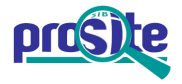Entry: PS00125

 Entry name [info] SER_THR_PHOSPHATASE Accession [info] PS00125 Entry type [info] PATTERN Date [info] 01-APR-1990 CREATED; 01-DEC-2001 DATA UPDATE; 17-JUN-2020 INFO UPDATE. PROSITE Doc. [info] PDOC00115

Name and characterization of the entry

 Description [info] Serine/threonine specific protein phosphatases signature. Pattern [info] ```[LIVMN]-[KR]-G-N-H-E. ```

Numerical results [info]

Numerical results for UniProtKB/Swiss-Prot release 2020_03 which contains 562'755 sequence entries.

 Total number of hits 211 in 211 different sequences Number of true positive hits 203 in 203 different sequences Number of 'unknown' hits 0 Number of false positive hits 8 in 8 different sequences Number of false negative sequences 5 Number of 'partial' sequences 1 Precision (true positives / (true positives + false positives)) 96.21 % Recall (true positives / (true positives + false negatives)) 97.60 %

 UniProtKB/Swiss-ProtTrue positive sequences 203 sequences ```BSL1_ARATH (Q8L7U5 ), BSL1_ORYSJ (Q60EX6 ), BSL2_ARATH (Q9SJF0 ), ``` ```BSL2_ORYSJ (Q2QM47 ), BSL3_ARATH (Q9SHS7 ), BSU1_ARATH (Q9LR78 ), FYPP1_ARATH (Q9SX52 ), FYPP3_ARATH (Q9LHE7 ), FYPP_PEA (Q8LSN3 ), GLC7A_CAEBR (Q61JR3 ), GLC7A_CAEEL (Q27497 ), GLC7B_CAEBR (Q627N3 ), GLC7B_CAEEL (P48727 ), GLC7C_CAEEL (O02658 ), GLC7D_CAEEL (P91420 ), PP11_ACEPE (P48480 ), PP11_ARATH (P30366 ), PP11_DROME (P48461 ), PP11_SCHPO (P13681 ), PP11_TOBAC (O04856 ), PP11_TRYBB (P23733 ), PP11_YEAST (P20604 ), PP12_ACEPE (P48481 ), PP12_ARATH (P48482 ), PP12_DROME (P12982 ), PP12_SCHPO (P23880 ), PP12_TOBAC (O04857 ), PP12_TRYBB (P23734 ), PP12_YEAST (P32598 ), PP13_ARATH (P48483 ), PP13_DROME (Q05547 ), PP13_TOBAC (O04858 ), PP14_ARATH (P48484 ), PP15_ARATH (P48485 ), PP16_ARATH (P48486 ), PP17_ARATH (O82733 ), PP18_ARATH (O82734 ), PP19_ARATH (Q9M9W3 ), PP1A_BOVIN (Q3T0E7 ), PP1A_CANLF (Q8WMS6 ), PP1A_HUMAN (P62136 ), PP1A_MOUSE (P62137 ), PP1A_RABIT (P62139 ), PP1A_RAT (P62138 ), PP1B_BOVIN (Q3SWW9 ), PP1B_CANLF (Q8MJ47 ), PP1B_CHICK (P62207 ), PP1B_DROME (P48462 ), PP1B_HUMAN (P62140 ), PP1B_MOUSE (P62141 ), PP1B_PIG (P61292 ), PP1B_PONAB (Q5R740 ), PP1B_RABIT (P62143 ), PP1B_RAT (P62142 ), PP1B_XENLA (Q6GQL2 ), PP1B_XENTR (Q5I085 ), PP1GA_XENLA (P36874 ), PP1GB_XENLA (Q7SZ10 ), PP1G_BOVIN (P61287 ), PP1G_CANLF (Q8MJ46 ), PP1G_HUMAN (P36873 ), PP1G_MOUSE (P63087 ), PP1G_RAT (P63088 ), PP1_BRANA (P23777 ), PP1_BRAOL (P48487 ), PP1_DICDI (O15757 ), PP1_EMENI (P20654 ), PP1_MAIZE (P22198 ), PP1_MEDSV (P48488 ), PP1_NEUCR (Q9UW86 ), PP1_ORYSJ (P48489 ), PP1_PHAVU (P48490 ), PP2A1_ARATH (Q07099 ), PP2A1_EMENI (Q9HFQ2 ), PP2A1_NEUCR (P48580 ), PP2A1_ORYSI (A2YEB4 ), PP2A1_ORYSJ (Q0DBD3 ), PP2A1_PARTE (P48726 ), PP2A1_SCHPO (P23635 ), PP2A1_YEAST (P23594 ), PP2A2_ARATH (Q07098 ), PP2A2_BLUGH (Q8X178 ), PP2A2_ORYSI (A2XN40 ), PP2A2_ORYSJ (Q10BT5 ), PP2A2_PARTE (A0CNL9 ), PP2A2_SCHPO (P23636 ), PP2A2_YEAST (P23595 ), PP2A3_ARATH (Q07100 ), PP2A3_ORYSI (A2X2G3 ), PP2A3_ORYSJ (Q0E2S4 ), PP2A3_PARTE (Q6BFF6 ), PP2A4_ARATH (P48578 ), PP2A4_ORYSI (P0C5D7 ), PP2A4_ORYSJ (A3C4N5 ), PP2A4_YEAST (P32838 ), PP2A5_ARATH (O04951 ), PP2A5_TOBAC (O04860 ), PP2AA_BOVIN (P67774 ), PP2AA_CHICK (P48463 ), PP2AA_DICDI (Q9XZE5 ), PP2AA_HUMAN (P67775 ), PP2AA_MOUSE (P63330 ), PP2AA_PIG (P67776 ), PP2AA_RABIT (P67777 ), PP2AA_RAT (P63331 ), PP2AB_BOVIN (Q0P594 ), PP2AB_DICDI (Q54RD6 ), PP2AB_HUMAN (P62714 ), PP2AB_MOUSE (P62715 ), PP2AB_PIG (P11493 ), PP2AB_RABIT (P11611 ), PP2AB_RAT (P62716 ), PP2A_ACEPE (P48577 ), PP2A_BRANA (P23778 ), PP2A_CAEEL (G5EGK8 ), PP2A_DROME (P23696 ), PP2A_HELAN (P48579 ), PP2A_HEVBR (Q9ZSE4 ), PP2A_MEDSA (Q06009 ), PP2A_TOBAC (Q9XGH7 ), PP2B1_CRYNH (O42773 ), PP2B1_DROME (P48456 ), PP2B1_YEAST (P23287 ), PP2B2_DROME (Q27889 ), PP2B2_YEAST (P14747 ), PP2B3_DROME (Q9VXF1 ), PP2BA_BOVIN (P48452 ), PP2BA_DICDI (Q7YSW8 ), PP2BA_HUMAN (Q08209 ), PP2BA_MOUSE (P63328 ), PP2BA_RAT (P63329 ), PP2BB_HUMAN (P16298 ), PP2BB_MOUSE (P48453 ), PP2BB_RAT (P20651 ), PP2BC_HUMAN (P48454 ), PP2BC_MOUSE (P48455 ), PP2B_ASPFU (Q4WUR1 ), PP2B_CAEEL (Q0G819 ), PP2B_EMENI (P48457 ), PP2B_NEUCR (Q05681 ), PP2B_SCHPO (Q12705 ), PP4C1_CAEBR (A8XE00 ), PP4C1_CAEEL (Q9XW79 ), PP4C2_CAEEL (Q9XTT8 ), PP4CA_DANRE (A8WGP3 ), PP4CB_DANRE (A9JRC7 ), PP4C_ASHGO (Q74ZR2 ), PP4C_BOVIN (A6H772 ), PP4C_CANGA (Q6FM81 ), PP4C_DICDI (Q9Y0B7 ), PP4C_DROME (O76932 ), PP4C_HUMAN (P60510 ), PP4C_KLULA (Q6CNT6 ), PP4C_MOUSE (P97470 ), PP4C_PONAB (Q5R6K8 ), PP4C_RABIT (P11084 ), PP4C_RAT (Q5BJ92 ), PP4C_XENLA (Q6IP91 ), PP4C_XENTR (Q6P861 ), PP4C_YEAST (P32345 ), PPE1_HUMAN (O14829 ), PPE1_MOUSE (O35655 ), PPE1_RAT (Q3SWT6 ), PPE1_SCHPO (P36614 ), PPE2_HUMAN (O14830 ), PPE2_MOUSE (O35385 ), PPE_CAEEL (G5EBX9 ), PPG1_CANAL (Q5A6B6 ), PPIG_XENTR (Q6NVU2 ), PPP5_HUMAN (P53041 ), PPP5_MOUSE (Q60676 ), PPP5_RAT (P53042 ), PPP6_CAEEL (Q09496 ), PPP6_DICDI (Q9U9A3 ), PPP6_DROME (Q27884 ), PPP6_HUMAN (O00743 ), PPP6_MOUSE (Q9CQR6 ), PPP6_RAT (Q64620 ), PPP7_ARATH (Q9FN02 ), PPQ1_YEAST (P32945 ), PPT1_SCHPO (O43049 ), PPT1_YEAST (P53043 ), PPX1_ARATH (P48529 ), PPX1_PARTE (P49576 ), PPX2_ARATH (P48528 ), PPX2_PARTE (A0DJ90 ), PPX3_PARTE (A0C1E4 ), PPX4_PARTE (A0CCD2 ), PPY_DROME (P11612 ), PPZ1_YEAST (P26570 ), PPZ2_YEAST (P33329 ), PPZ_SCHPO (P78968 ), PP_LAMBD (P03772 ), PRP1_ECOLI (P55798 ), PRP1_SALTY (Q8ZNY9 ), PRP2_ECOLI (P55799 ), RDGC_DROME (P40421 ), SIT4_CANAL (Q59KY8 ), YD44_SCHPO (Q10298 ), YOU5_SCHPO (O74789 ), YSD1_CAEEL (P48459 ), YT91_CAEEL (P48458 ), YY06_CAEEL (P48460 ) ``` » more Retrieve an alignment of UniProtKB/Swiss-Prot true positive hits: [Clustal format, color, condensed view] [Clustal format, color] [Clustal format, plain text] [Fasta format] Retrieve the sequence logo from the alignment UniProtKB/Swiss-ProtFalse negative sequences 5 sequences ```PPP5_ARATH (Q84XU2 ), PPP5_SOLLC (Q84K11 ), PPP7I_ARATH (Q9LEV0 ), ``` ```PPP7L_ARATH (Q9LNG5 ), PRP2_SALTY (Q8ZMH3 ) ``` » more UniProtKB/Swiss-Prot'Partial' sequences 1 sequence ```PPP5_RABIT (P55739 ) ``` UniProtKB/Swiss-ProtFalse positive sequences 8 sequences ```GFD1_YEAST (Q04839 ), LAP1_CHAGB (Q2H1T8 ), LAP1_NEUCR (Q7RYC8 ), ``` ```NFM_CHICK (P16053 ), RPM1_CAEEL (Q17551 ), SYP_PELCD (Q3A6R6 ), TASO2_MOUSE (Q5DTT3 ), XIRP2_MOUSE (Q4U4S6 ) ``` » more